# Digital logic test questions for interview, exams, entrance

## Digital logic test questions for interview, exams, entrance

(1) For the function f (w, x, y, z) = ∑ (0, 4, 5, 7, 8, 9, 13, 15) the expression which is pre not equivalent to f is

(A) x’y’z’ + wx’y’ + w’y
(B) xyz + wx’y’ + w’y
(C) x’y’z’ + wy’ + w’yz + wz
(D) x’yz’ + x’y’ + w’y + xz

ANSWER: x’y’z’ + wx’y’ + w’y

(2) The initial value of the output Q1Q0 for the sequential circuit shown below is 00. The next four values of Q1Q0 is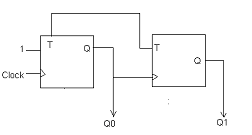(A) 01, 10, 01, 10
(B) 11, 11, 00, 10
(C) 11, 10, 01, 00
(D) 00, 10, 10, 10

(3) The finite state machine shown below takes binary input from the least significant bit. The statement that holds true is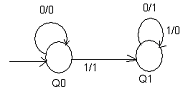(A) 1’s compliment of the input number is computed
(B) 2’s compliment of the input number is computed
(C) The input number is decremented
(D) The input number is increment

ANSWER: 2’s compliment of the input number is computed

(4) The behavior of 1-input, 2-output synchronous sequential circuit is given below:

In the initial k bits of the input, number of 0’s is represented by zk and number of 1’s is represented by nk.
(zk + nk = k). The output of the circuit is 00 only if one of the conditions given below is correct.
Case 1. zk – nk = 2, the output at the kth and all subsequent clock ticks is 10.
Case 2. nk – zk = 2, the output at the kth and all subsequent clock ticks is 01.

In the state transition graph of the above circuit the minimum number of states required is

(A) 6
(B) 5
(C) 8
(D) 7

(5) For the number (-539)10 the 2’s compliment representation in hexadecimal form is

(A) DE5
(B) DBC
(C) 9D6
(D) ABC

(6) Consider the karnaugh map shown below where X denotes “don’t care”. The literal count of a Boolean expression is defined as the sum of the number of times each literal appears in the expression. For example, the literal count of (xy + xz) is 4. The minimum possible literal counts of the POS and SOP representations respectively of the function given by the Karnaugh map is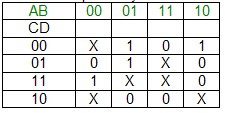(A) (9, 10)
(B) (10, 9)
(C) (11, 11)
(D) (12, 13)

(7) What is the 2’s compliment representation of the number 43?

(A) 11000110
(B) 00101011
(C) 01011101
(D) 10010010

(8) For the circuit shown below the statement that holds true is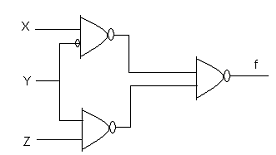(A) f is independent of X.
(B) f is independent of Y.
(C) f is independent of Z.
(D) None of X, Y, Z is redundant.

ANSWER: None of X, Y, Z is redundant.

(9) The function that is not implemented by the karnaugh map shown below is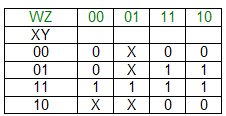(A) XY + YW
(B) (W + X)(W + Y)(X + Y)
(C) (W + Y) X
(D) None of the above

(10) A = 2.0 x 1030 , B = -2.0 x 1030 and C = 1.0. Consider the sequence

X: = A + B
Y: = A + C
X: = X + C
Y: = Y + B

What is the value of X and Y?

(A) X = Y = 0.0
(B) X = 0.0, Y = 1.0
(C) X = 1.0, Y = 0.0
(D) X = Y = 1.0

ANSWER: X = Y = 1.0

(11) The function that implements karnaugh map shown below is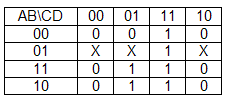(A) (C + D)(C’ + D)(A + B)
(B) D(C + A)
(C) A’B + CD

(12) What is the minterm expansion of f (P, Q, R) = PQ + QR’ + PR’ ?

(A) m2 + m3 + m0 + m6
(B) m2 + m0 + m3 + m1
(C) m2 + m4 + m6 + m1
(D) m0 + m4 + m6 + m1

ANSWER: m2 + m4 + m6 + m1

(13) The state table for the sequential machine is shown below: The number of states in the minimized machine will be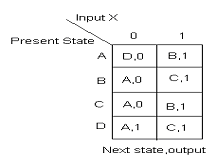(A) 1
(B) 2
(C) 3
(D) 4

(14) For the decimal number –57 Booth’s coding in 8 bits is

(A) 0 – 100 + 100 - 1
(B) 0 – 100 + 1000
(C) 00 – 10 +100 - 1
(D) 0 – 1 + 100 - 1

ANSWER: 0 – 100 + 100 - 1

(15) Consider the truth table given below: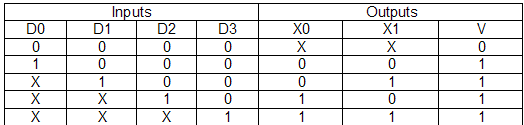If the input is valid then only V = 1. The truth table represents

(A) Demultiplexer
(B) Multiplexer
(C) Decoder
(D) Priority encoder

(16) When the multiplier pattern is ________________ Booth’s algorithm for integer multiplication gives worst performance.

(A) 011111……..11110
(B) 101010………1010
(C) 100000…..001
(D) 111111…….1111

(17) Zero has two representations in

(A) 1’s compliment
(B) Sign magnitude
(C) Both A and B
(D) Neither A nor B

(18) The figure shows the arrangement of master slave flip-flops. The initial state of P is 0 and that of Q is 1. The output state P and Q after the clock cycles is respectively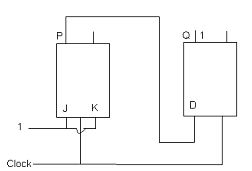(A) 0, 0
(B) 0, 1
(C) 1, 1
(D) 1, 0

(19) To implement any arbitrary Boolean function the set of components that are sufficient is

(A) AND gate and NOT gate
(B) AND gate and XOR gate
(C) 2 to 1 multiplexer
(D) XOR gate and NOT gate

ANSWER: AND gate and XOR gate

(20) The decimal value 05 in IEEE single precision floating-point representation has

(A) Fraction bits of 100….000 and exponent value of 0
(B) Fraction bits of 000….000 and exponent value of -1
(C) Fraction bits of 000….000 and exponent value of 0
(D) No exact representation

ANSWER: Fraction bits of 100….000 and exponent value of 0

(21) The output of a 2:1 MUX is given by the function (ac’ + bc). The statement that holds true is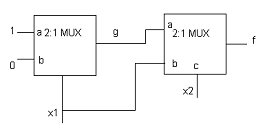(A) f = x1 + x2
(B) f = x1x2 + x1’x2’
(C) f = x1 + x2’
(D) f = x1’ + x2

ANSWER: f = x1x2 + x1’x2’

(22) The simultaneous equations are given below:

x + y + z = 1
xy = 0
xz + w = 1
xy + z’w’ = 0

The values of x, y, z and w are respectively

(A) 1 0 1 1
(B) 1 0 0 0
(C) 1 1 0 0
(D) 0 1 1 0

(23) What is the simplified expression for the function f (f (x + y, y), z) if f (A, B) = A’ + B?

(A) x + y’
(B) x’ + z
(C) xyz
(D) None of the above

(24) AND, OR, NOT, NAND and NOR gates are used to design a 4-bit carry look ahead adder which adds two 4-bit numbers. The delay of each gate is 1 time unit. The carry network has been implemented using two-level AND-OR logic and all the inputs are available in both complemented and uncomplemented form. The overall propagation delay of the adder is

(A) 3 time unit
(B) 15 time unit
(C) 10 time unit
(D) 6 time unit

(25) The output of the circuit is in the form of 4-bit. The output 0 is represented by 0000, 1 by 0001… 9 by 1001. If we design a combinational circuit which takes these 4 bits as input the output will be 1 if the digit = 5 and otherwise. Only AND, OR and NOT gates are to be used. To design this circuit the minimum number of gates required is

(A) 2
(B) 3
(C) 4
(D) 5

(26) Two 8 bit 2’s compliment number’s are 1111 1010 and 0000 1010. Their product in 2’s compliment is

(A) 1100 0100
(B) 1010 0010
(C) 1100 1100
(D) 1000 1111

(27) For the Boolean function f (a, b, c) = a’c + ac’ + b’c the essential prime implicants are

(A) ac’ and bc’
(B) a’c only
(C) a’c and b’c
(D) a’c and ac’

(28) Sign extension is a step in

(B) Arithmetic left shift
(C) Converting a signed integer from one size to another
(D) Floating point multiplication

For questions 29 and 30 refer to the data given below:

The floating-point format is given below whose mantissa is a pure fraction in sign-magnitude form: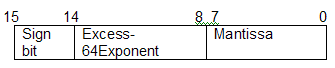(29) The hexadecimal representation (without normalization and rounding off) of the decimal number 0.239 x 213 is

(A) 4D0D
(B) 4D3D
(C) 0D24
(D) 0D4D

(30) For the above format the normalized representation is specified as follows:

The mantissa has an implicit preceding the binary (radix) point. Assuming that only 0’s are padded in while shifting a field the normalized representation of the above number 0.239 x

213 is

(A) D0D0
(B) 4AE8
(C) 49D0
(D) D048

(31) Consider a number n whose 4-bit gray code representation is a3a2a1a0 and the gray code of (n + 1) (module 16) is b3b2b1b0. The function, which is correct, is

(A) b3 (a3a2a1a0) = ∑ (0, 1, 6, 7, 10, 13, 14, 15)
(B) b2 (a3a2a1a0) = ∑ (2, 4, 5, 6, 10, 13, 14, 15)
(C) b1 (a3a2a1a0) = ∑ (4, 9, 10, 11, 12, 13, 14, 15)
(D) b0 (a3a2a1a0) = ∑ (1, 2, 3, 6, 10, 13, 14, 15)

ANSWER: b1 (a3a2a1a0) = ∑ (4, 9, 10, 11, 12, 13, 14, 15)

(32) For the decimal value –15 the 2’s compliment representation is

(A) 10001
(B) 11100
(C) 11010
(D) 11111

(33) We have the Boolean expression X * Y = XY + X’Y’ and Z = X * Y. Which of the following is a valid expression?

(A) X = Y * Z
(B) Y = X * Z
(C) X * Y * Z = 1
(D) All of the above

(34) What is the minimum size of multiplexer needed to implement any Boolean function of n variables if we are allowed to use only one multiplexer and one inverter?

(A) 2n-2 line to 1 line
(B) 2n+1 line to 1 line
(C) 2n line to 1 line
(D) 2n-1 line to 1 line

ANSWER: 2n-1 line to 1 line

(35) Which one of the following statement hold true for the decimal value 0.25?

(A) It cannot be represented precisely in binary
(B) It is equivalent to binary value 0.001
(C) It is equivalent to binary value 0.01
(D) It is equivalent to binary value 0.1

ANSWER: It is equivalent to binary value 0.01

(36) What is the value of x and y if 73x = 54y

(A) 10, 12
(B) 8, 11
(C) 9, 13
(D) None of the above

(37) We have the function with four variables f (A, B, C, D) = ∑ (1, 4, 5, 9, 11, 12). The switching expression corresponding to this function is

(A) BC’D’ + A’C’D + AB’D
(B) ABC’ + BCD’ + AB’D
(C) A’B’C’ + A’CD + AC’D’
(D) ACD’ + A’B’C + ABD

ANSWER: BC’D’ + A’C’D + AB’D

(38) The two expressions that are given below is evaluated using three digit floating point arithmetic with rounding:

(113 + - 111) + 7.51
113 + (-111 + 7.51)

The result will be

(A) 10.0 and 9.51
(B) 10.0 and 10.0
(C) 9.51 and 10.0
(D) 9.51 and 9.51

(39) By cross-coupling two NAND gates an SR latch is made. If the inputs S = R = 0 then the output will be

(A) Q = 1, Q’ = 1
(B) Q = 0, Q’ = 1
(C) Q = 1, Q’ = 0
(D) Indeterminate states

(40) If we assume that all the numbers are in 2’s compliment representation then the number which is divisible by 11111011 is

(A) 11011011
(B) 11100111
(C) 11010111
(D) 11100100

(41) A Boolean function x’y’ + xy + x’y is equivalent to

(A) x’ + y’
(B) x + y’
(C) x’ + y
(D) x + y

(42) The function with four variables f (a, b, c, d) = ∑ (1, 3, 4, 6, 9, 11, 12, 14 is

(A) Independent of two variables
(B) Independent of one variable
(C) Independent of three variables
(D) Dependent on all the variables

(43) Which one of the following is the hexadecimal representation of 6578?

(A) 108
(B) 32F
(C) D78
(D) 1AF

(44) Which one of the following is equivalent to (1217) 8?

(A) (1217) 16
(B) (2424) 16
(C) (028F) 16
(D) (0B25) 16

(45) If we have n number of Boolean variables then the maximum number of different Boolean functions involving n is

(A) nn
(B) 2n
(C) n2
(D) None of the above

(46) Which one of the following range of integers can be represented by an n bit 2’s complement number system?

(A) -(2n-1 -1) to (2n-1 -1)
(B) -2n-1 to (2n-1 –1)
(C) -(2n-1 +1) to (2n-1 -1)
(D) -2n-1 to 2n-1

(47) We have the hexadecimal value 0 x 00000000 in the IEEE floating point representation. This will correspond to

(A) The special value +0
(B) The normalized value 2-127
(C) The normalized value 2-256
(D) The normalized value +0

(48) We need to construct 6-to-64 line decoder without using any other logic gates. How many 3-to-8 line decoders wit an enable input are needed for the same?

(A) 11
(B) 8
(C) 15
(D) 9

(49) If p denotes the number system radix then the only value of p that satisfy the equation √121p =

11p is

(A) Any value >2
(B) Any value <2
(C) Decimal 10
(D) None of the above

(50) The 2’s complement representation of P, which is a 16-bit signed integer, is (F87B)16. What is the 2s ’complement representation of 8*P?

(A) (1870) 16
(B) (C3D8) 16
(C) (ACD8) 16
(D) (C103) 16

(51) The Boolean function (AB+C) is to be implemented using only 2-input NOR gates. The minimum number of gates required is

(A) 5
(B) 4
(C) 3
(D) 2

(52) How many D flip-flops are needed to design a mod-258 counter?

(A) 121
(B) 5
(C) 258
(D) 9